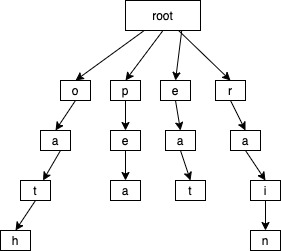Given a 2D board and a list of words from the dictionary, find all words in the board. Each word must be constructed from letters of sequentially adjacent cell, where “adjacent” cells are those horizontally or vertically neighboring. The same letter cell may not be used more than once in a word.

Note:

• All inputs are consist of lowercase letters a-z.
• The values of words are distinct.

Example:

``````Input:
board = [
['o','a','a','n'],
['e','t','a','e'],
['i','h','k','r'],
['i','f','l','v']
]
words = ["oath","pea","eat","rain"]

Output: ["eat","oath"]
``````

# Solution

In order to solve this problem we can apply the Trie data structure and DFS (Depth First Search) algorithm. Trie can be applied to the boards itself as well as to the given words. Lets go with approach of building a trie for the given wordsThe code for building a Trie goes as follows:

``````private void buildTrie(TrieNode root, String[] words) {
for (String word : words) {
TrieNode cur = root;
for (char ch : word.toCharArray()) {
int index = (int)(ch - 'a');
if (cur.children[index] == null) {
cur.children[index] = new TrieNode();
}
cur = cur.children[index];
}
cur.word = word;
}
}
``````

After that we start scanning each cell of the board and try to DFS search trough it and try to match with our trie. While DFS searching through the board we have to mark already visited cells to avoid repetition (Yeah actually if we don’t do it we will be cycling there forever). Lets use the ‘#’ sign as already visited signature. Of course before marking it with ‘#’ (already visited sign) we have to remember the original value of the cell. That way we can put it back when we are done with dfs scanning.

This is how the board will be look like while we are scanning and when we found the word ‘oath’

``````  ['#','#','a','n']
['e','#','a','e']
['i','#','k','r']
['i','f','l','v']
``````

Remembering the board values happens in each level of recursion, so we don’t have to worry about it. Here how the code will look like DFS recursion.

``````private void dfs(char[][] board, int row, int col, TrieNode cur, List<String> result) {
// border checkings
if (row < 0 || col < 0
|| row > board.length - 1
|| col > board.length - 1) return;

// if already visited then get out
if (board[row][col] == '#') return;

// get the board value and remeber it in c
char c = board[row][col];

// if there is no child with current cell character then get out
if (cur.children[c - 'a'] == null) return;
cur = cur.children[c - 'a'];

// if the current child is flagged as a word then we found a word
if (cur.word != null) {
cur.word = null;
}

// marking the cell as visited
board[row][col] = '#';

// lets scan in four directions
dfs(board, row + 1, col, cur, result);
dfs(board, row - 1, col, cur, result);
dfs(board, row, col + 1, cur, result);
dfs(board, row, col - 1, cur, result);

// putting back the remembered value of the board cell
board[row][col] = c;
}
``````

# Final Solution Code

``````class Solution {

class TrieNode {
String word;
TrieNode [] children;
TrieNode() {
children = new TrieNode;
word = null;
}
}

public List<String> findWords(char[][] board, String[] words) {
List<String> result = new ArrayList<>();
if (board == null || board.length == 0) return result;

TrieNode root = new TrieNode();
buildTrie(root, words);

for (int i = 0; i < board.length; i++) {
for (int j = 0; j < board.length; j++) {
dfs(board, i, j, root, result);
}
}

return result;
}

private void dfs(char[][] board, int row, int col, TrieNode cur, List<String> result) {
if (row < 0 || col < 0
|| row > board.length - 1
|| col > board.length - 1) return;

if (board[row][col] == '#') return;

char c = board[row][col];
if (cur.children[c - 'a'] == null) return;
cur = cur.children[c - 'a'];

if (cur.word != null) {
cur.word = null;
}

board[row][col] = '#';
dfs(board, row + 1, col, cur, result);
dfs(board, row - 1, col, cur, result);
dfs(board, row, col + 1, cur, result);
dfs(board, row, col - 1, cur, result);

board[row][col] = c;

}

private void buildTrie(TrieNode root, String[] words) {
for (String word : words) {
TrieNode cur = root;
for (char ch : word.toCharArray()) {
int index = (int)(ch - 'a');
if (cur.children[index] == null) {
cur.children[index] = new TrieNode();
}
cur = cur.children[index];
}
cur.word = word;
}
}
}
``````

Ref